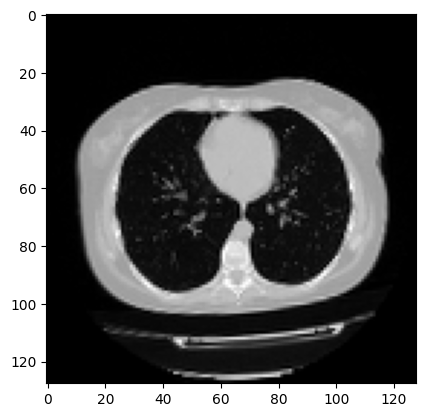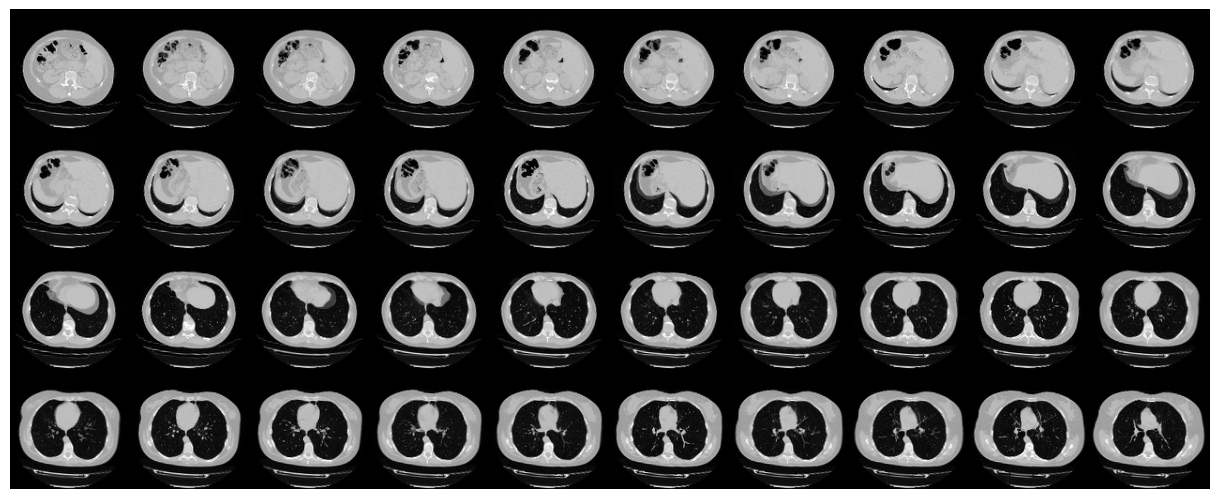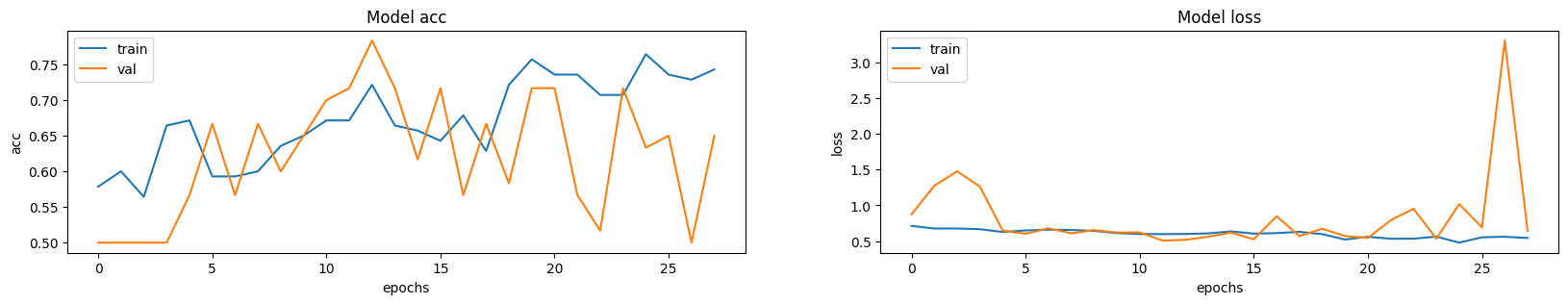» Code examples / Computer Vision / 3D image classification from CT scans

# 3D image classification from CT scans

Author: Hasib Zunair
Date created: 2020/09/23
Description: Train a 3D convolutional neural network to predict presence of pneumonia.View in ColabGitHub source

## Introduction

This example will show the steps needed to build a 3D convolutional neural network (CNN) to predict the presence of viral pneumonia in computer tomography (CT) scans. 2D CNNs are commonly used to process RGB images (3 channels). A 3D CNN is simply the 3D equivalent: it takes as input a 3D volume or a sequence of 2D frames (e.g. slices in a CT scan), 3D CNNs are a powerful model for learning representations for volumetric data.

## Setup

``````import os
import zipfile
import numpy as np
import tensorflow as tf

from tensorflow import keras
from tensorflow.keras import layers
``````

In this example, we use a subset of the MosMedData: Chest CT Scans with COVID-19 Related Findings. This dataset consists of lung CT scans with COVID-19 related findings, as well as without such findings.

We will be using the associated radiological findings of the CT scans as labels to build a classifier to predict presence of viral pneumonia. Hence, the task is a binary classification problem.

``````# Download url of normal CT scans.
filename = os.path.join(os.getcwd(), "CT-0.zip")
keras.utils.get_file(filename, url)

filename = os.path.join(os.getcwd(), "CT-23.zip")
keras.utils.get_file(filename, url)

# Make a directory to store the data.
os.makedirs("MosMedData")

# Unzip data in the newly created directory.
with zipfile.ZipFile("CT-0.zip", "r") as z_fp:
z_fp.extractall("./MosMedData/")

with zipfile.ZipFile("CT-23.zip", "r") as z_fp:
z_fp.extractall("./MosMedData/")
``````
``````Downloading data from https://github.com/hasibzunair/3D-image-classification-tutorial/releases/download/v0.2/CT-0.zip
1065476096/1065471431 [==============================] - 236s 0us/step
271171584/1045162547 [======>.......................] - ETA: 2:56
``````

The files are provided in Nifti format with the extension .nii. To read the scans, we use the `nibabel` package. You can install the package via `pip install nibabel`. CT scans store raw voxel intensity in Hounsfield units (HU). They range from -1024 to above 2000 in this dataset. Above 400 are bones with different radiointensity, so this is used as a higher bound. A threshold between -1000 and 400 is commonly used to normalize CT scans.

To process the data, we do the following:

• We first rotate the volumes by 90 degrees, so the orientation is fixed
• We scale the HU values to be between 0 and 1.
• We resize width, height and depth.

Here we define several helper functions to process the data. These functions will be used when building training and validation datasets.

``````import nibabel as nib

from scipy import ndimage

# Get raw data
scan = scan.get_fdata()
return scan

def normalize(volume):
"""Normalize the volume"""
min = -1000
max = 400
volume[volume < min] = min
volume[volume > max] = max
volume = (volume - min) / (max - min)
volume = volume.astype("float32")
return volume

def resize_volume(img):
"""Resize across z-axis"""
# Set the desired depth
desired_depth = 64
desired_width = 128
desired_height = 128
# Get current depth
current_depth = img.shape[-1]
current_width = img.shape
current_height = img.shape
# Compute depth factor
depth = current_depth / desired_depth
width = current_width / desired_width
height = current_height / desired_height
depth_factor = 1 / depth
width_factor = 1 / width
height_factor = 1 / height
# Rotate
img = ndimage.rotate(img, 90, reshape=False)
# Resize across z-axis
img = ndimage.zoom(img, (width_factor, height_factor, depth_factor), order=1)
return img

def process_scan(path):
# Normalize
volume = normalize(volume)
# Resize width, height and depth
volume = resize_volume(volume)
return volume
``````

Let's read the paths of the CT scans from the class directories.

``````# Folder "CT-0" consist of CT scans having normal lung tissue,
# no CT-signs of viral pneumonia.
normal_scan_paths = [
os.path.join(os.getcwd(), "MosMedData/CT-0", x)
for x in os.listdir("MosMedData/CT-0")
]
# Folder "CT-23" consist of CT scans having several ground-glass opacifications,
# involvement of lung parenchyma.
abnormal_scan_paths = [
os.path.join(os.getcwd(), "MosMedData/CT-23", x)
for x in os.listdir("MosMedData/CT-23")
]

print("CT scans with normal lung tissue: " + str(len(normal_scan_paths)))
print("CT scans with abnormal lung tissue: " + str(len(abnormal_scan_paths)))
``````
``````CT scans with normal lung tissue: 100
CT scans with abnormal lung tissue: 100
``````

## Build train and validation datasets

Read the scans from the class directories and assign labels. Downsample the scans to have shape of 128x128x64. Rescale the raw HU values to the range 0 to 1. Lastly, split the dataset into train and validation subsets.

``````# Read and process the scans.
# Each scan is resized across height, width, and depth and rescaled.
abnormal_scans = np.array([process_scan(path) for path in abnormal_scan_paths])
normal_scans = np.array([process_scan(path) for path in normal_scan_paths])

# For the CT scans having presence of viral pneumonia
# assign 1, for the normal ones assign 0.
abnormal_labels = np.array([1 for _ in range(len(abnormal_scans))])
normal_labels = np.array([0 for _ in range(len(normal_scans))])

# Split data in the ratio 70-30 for training and validation.
x_train = np.concatenate((abnormal_scans[:70], normal_scans[:70]), axis=0)
y_train = np.concatenate((abnormal_labels[:70], normal_labels[:70]), axis=0)
x_val = np.concatenate((abnormal_scans[70:], normal_scans[70:]), axis=0)
y_val = np.concatenate((abnormal_labels[70:], normal_labels[70:]), axis=0)
print(
"Number of samples in train and validation are %d and %d."
% (x_train.shape, x_val.shape)
)
``````
``````Number of samples in train and validation are 140 and 60.
``````

## Data augmentation

The CT scans also augmented by rotating at random angles during training. Since the data is stored in rank-3 tensors of shape `(samples, height, width, depth)`, we add a dimension of size 1 at axis 4 to be able to perform 3D convolutions on the data. The new shape is thus `(samples, height, width, depth, 1)`. There are different kinds of preprocessing and augmentation techniques out there, this example shows a few simple ones to get started.

``````import random

from scipy import ndimage

@tf.function
def rotate(volume):
"""Rotate the volume by a few degrees"""

def scipy_rotate(volume):
# define some rotation angles
angles = [-20, -10, -5, 5, 10, 20]
# pick angles at random
angle = random.choice(angles)
# rotate volume
volume = ndimage.rotate(volume, angle, reshape=False)
volume[volume < 0] = 0
volume[volume > 1] = 1
return volume

augmented_volume = tf.numpy_function(scipy_rotate, [volume], tf.float32)
return augmented_volume

def train_preprocessing(volume, label):
"""Process training data by rotating and adding a channel."""
# Rotate volume
volume = rotate(volume)
volume = tf.expand_dims(volume, axis=3)
return volume, label

def validation_preprocessing(volume, label):
"""Process validation data by only adding a channel."""
volume = tf.expand_dims(volume, axis=3)
return volume, label
``````

While defining the train and validation data loader, the training data is passed through and augmentation function which randomly rotates volume at different angles. Note that both training and validation data are already rescaled to have values between 0 and 1.

``````# Define data loaders.

batch_size = 2
# Augment the on the fly during training.
train_dataset = (
.map(train_preprocessing)
.batch(batch_size)
.prefetch(2)
)
# Only rescale.
validation_dataset = (
.map(validation_preprocessing)
.batch(batch_size)
.prefetch(2)
)
``````

Visualize an augmented CT scan.

``````import matplotlib.pyplot as plt

data = train_dataset.take(1)
images, labels = list(data)
images = images.numpy()
image = images
print("Dimension of the CT scan is:", image.shape)
plt.imshow(np.squeeze(image[:, :, 30]), cmap="gray")
``````
``````Dimension of the CT scan is: (128, 128, 64, 1)

<matplotlib.image.AxesImage at 0x7fea680354e0>
``````Since a CT scan has many slices, let's visualize a montage of the slices.

``````def plot_slices(num_rows, num_columns, width, height, data):
"""Plot a montage of 20 CT slices"""
data = np.rot90(np.array(data))
data = np.transpose(data)
data = np.reshape(data, (num_rows, num_columns, width, height))
rows_data, columns_data = data.shape, data.shape
heights = [slc.shape for slc in data]
widths = [slc.shape for slc in data]
fig_width = 12.0
fig_height = fig_width * sum(heights) / sum(widths)
f, axarr = plt.subplots(
rows_data,
columns_data,
figsize=(fig_width, fig_height),
gridspec_kw={"height_ratios": heights},
)
for i in range(rows_data):
for j in range(columns_data):
axarr[i, j].imshow(data[i][j], cmap="gray")
axarr[i, j].axis("off")
plt.subplots_adjust(wspace=0, hspace=0, left=0, right=1, bottom=0, top=1)
plt.show()

# Visualize montage of slices.
# 4 rows and 10 columns for 100 slices of the CT scan.
plot_slices(4, 10, 128, 128, image[:, :, :40])
``````## Define a 3D convolutional neural network

To make the model easier to understand, we structure it into blocks. The architecture of the 3D CNN used in this example is based on this paper.

``````def get_model(width=128, height=128, depth=64):
"""Build a 3D convolutional neural network model."""

inputs = keras.Input((width, height, depth, 1))

x = layers.Conv3D(filters=64, kernel_size=3, activation="relu")(inputs)
x = layers.MaxPool3D(pool_size=2)(x)
x = layers.BatchNormalization()(x)

x = layers.Conv3D(filters=64, kernel_size=3, activation="relu")(x)
x = layers.MaxPool3D(pool_size=2)(x)
x = layers.BatchNormalization()(x)

x = layers.Conv3D(filters=128, kernel_size=3, activation="relu")(x)
x = layers.MaxPool3D(pool_size=2)(x)
x = layers.BatchNormalization()(x)

x = layers.Conv3D(filters=256, kernel_size=3, activation="relu")(x)
x = layers.MaxPool3D(pool_size=2)(x)
x = layers.BatchNormalization()(x)

x = layers.GlobalAveragePooling3D()(x)
x = layers.Dense(units=512, activation="relu")(x)
x = layers.Dropout(0.3)(x)

outputs = layers.Dense(units=1, activation="sigmoid")(x)

# Define the model.
model = keras.Model(inputs, outputs, name="3dcnn")
return model

# Build model.
model = get_model(width=128, height=128, depth=64)
model.summary()
``````
``````Model: "3dcnn"
_________________________________________________________________
Layer (type)                 Output Shape              Param #
=================================================================
input_1 (InputLayer)         [(None, 128, 128, 64, 1)] 0
_________________________________________________________________
conv3d (Conv3D)              (None, 126, 126, 62, 64)  1792
_________________________________________________________________
max_pooling3d (MaxPooling3D) (None, 63, 63, 31, 64)    0
_________________________________________________________________
batch_normalization (BatchNo (None, 63, 63, 31, 64)    256
_________________________________________________________________
conv3d_1 (Conv3D)            (None, 61, 61, 29, 64)    110656
_________________________________________________________________
max_pooling3d_1 (MaxPooling3 (None, 30, 30, 14, 64)    0
_________________________________________________________________
batch_normalization_1 (Batch (None, 30, 30, 14, 64)    256
_________________________________________________________________
conv3d_2 (Conv3D)            (None, 28, 28, 12, 128)   221312
_________________________________________________________________
max_pooling3d_2 (MaxPooling3 (None, 14, 14, 6, 128)    0
_________________________________________________________________
batch_normalization_2 (Batch (None, 14, 14, 6, 128)    512
_________________________________________________________________
conv3d_3 (Conv3D)            (None, 12, 12, 4, 256)    884992
_________________________________________________________________
max_pooling3d_3 (MaxPooling3 (None, 6, 6, 2, 256)      0
_________________________________________________________________
batch_normalization_3 (Batch (None, 6, 6, 2, 256)      1024
_________________________________________________________________
global_average_pooling3d (Gl (None, 256)               0
_________________________________________________________________
dense (Dense)                (None, 512)               131584
_________________________________________________________________
dropout (Dropout)            (None, 512)               0
_________________________________________________________________
dense_1 (Dense)              (None, 1)                 513
=================================================================
Total params: 1,352,897
Trainable params: 1,351,873
Non-trainable params: 1,024
_________________________________________________________________
``````

## Train model

``````# Compile model.
initial_learning_rate = 0.0001
lr_schedule = keras.optimizers.schedules.ExponentialDecay(
initial_learning_rate, decay_steps=100000, decay_rate=0.96, staircase=True
)
model.compile(
loss="binary_crossentropy",
metrics=["acc"],
)

# Define callbacks.
checkpoint_cb = keras.callbacks.ModelCheckpoint(
"3d_image_classification.h5", save_best_only=True
)
early_stopping_cb = keras.callbacks.EarlyStopping(monitor="val_acc", patience=15)

# Train the model, doing validation at the end of each epoch
epochs = 100
model.fit(
train_dataset,
validation_data=validation_dataset,
epochs=epochs,
shuffle=True,
verbose=2,
callbacks=[checkpoint_cb, early_stopping_cb],
)
``````
``````Epoch 1/100
70/70 - 12s - loss: 0.7031 - acc: 0.5286 - val_loss: 1.1421 - val_acc: 0.5000
Epoch 2/100
70/70 - 12s - loss: 0.6769 - acc: 0.5929 - val_loss: 1.3491 - val_acc: 0.5000
Epoch 3/100
70/70 - 12s - loss: 0.6543 - acc: 0.6286 - val_loss: 1.5108 - val_acc: 0.5000
Epoch 4/100
70/70 - 12s - loss: 0.6236 - acc: 0.6714 - val_loss: 2.5255 - val_acc: 0.5000
Epoch 5/100
70/70 - 12s - loss: 0.6628 - acc: 0.6000 - val_loss: 1.8446 - val_acc: 0.5000
Epoch 6/100
70/70 - 12s - loss: 0.6621 - acc: 0.6071 - val_loss: 1.9661 - val_acc: 0.5000
Epoch 7/100
70/70 - 12s - loss: 0.6346 - acc: 0.6571 - val_loss: 2.8997 - val_acc: 0.5000
Epoch 8/100
70/70 - 12s - loss: 0.6501 - acc: 0.6071 - val_loss: 1.6101 - val_acc: 0.5000
Epoch 9/100
70/70 - 12s - loss: 0.6065 - acc: 0.6571 - val_loss: 0.8688 - val_acc: 0.6167
Epoch 10/100
70/70 - 12s - loss: 0.5970 - acc: 0.6714 - val_loss: 0.8802 - val_acc: 0.5167
Epoch 11/100
70/70 - 12s - loss: 0.5910 - acc: 0.7143 - val_loss: 0.7282 - val_acc: 0.6333
Epoch 12/100
70/70 - 12s - loss: 0.6147 - acc: 0.6500 - val_loss: 0.5828 - val_acc: 0.7500
Epoch 13/100
70/70 - 12s - loss: 0.5641 - acc: 0.7214 - val_loss: 0.7080 - val_acc: 0.6667
Epoch 14/100
70/70 - 12s - loss: 0.5664 - acc: 0.6857 - val_loss: 0.5641 - val_acc: 0.7000
Epoch 15/100
70/70 - 12s - loss: 0.5924 - acc: 0.6929 - val_loss: 0.7595 - val_acc: 0.6000
Epoch 16/100
70/70 - 12s - loss: 0.5389 - acc: 0.7071 - val_loss: 0.5719 - val_acc: 0.7833
Epoch 17/100
70/70 - 12s - loss: 0.5493 - acc: 0.6714 - val_loss: 0.5234 - val_acc: 0.7500
Epoch 18/100
70/70 - 12s - loss: 0.5050 - acc: 0.7786 - val_loss: 0.7359 - val_acc: 0.6000
Epoch 19/100
70/70 - 12s - loss: 0.5152 - acc: 0.7286 - val_loss: 0.6469 - val_acc: 0.6500
Epoch 20/100
70/70 - 12s - loss: 0.5015 - acc: 0.7786 - val_loss: 0.5651 - val_acc: 0.7333
Epoch 21/100
70/70 - 12s - loss: 0.4975 - acc: 0.7786 - val_loss: 0.8707 - val_acc: 0.5500
Epoch 22/100
70/70 - 12s - loss: 0.4470 - acc: 0.7714 - val_loss: 0.5577 - val_acc: 0.7500
Epoch 23/100
70/70 - 12s - loss: 0.5489 - acc: 0.7071 - val_loss: 0.9929 - val_acc: 0.6500
Epoch 24/100
70/70 - 12s - loss: 0.5045 - acc: 0.7357 - val_loss: 0.5891 - val_acc: 0.7333
Epoch 25/100
70/70 - 12s - loss: 0.5598 - acc: 0.7500 - val_loss: 0.5703 - val_acc: 0.7667
Epoch 26/100
70/70 - 12s - loss: 0.4822 - acc: 0.7429 - val_loss: 0.5631 - val_acc: 0.7333
Epoch 27/100
70/70 - 12s - loss: 0.5572 - acc: 0.7000 - val_loss: 0.6255 - val_acc: 0.6500
Epoch 28/100
70/70 - 12s - loss: 0.4694 - acc: 0.7643 - val_loss: 0.7007 - val_acc: 0.6833
Epoch 29/100
70/70 - 12s - loss: 0.4870 - acc: 0.7571 - val_loss: 1.7148 - val_acc: 0.5667
Epoch 30/100
70/70 - 12s - loss: 0.4794 - acc: 0.7500 - val_loss: 0.5744 - val_acc: 0.7333
Epoch 31/100
70/70 - 12s - loss: 0.4632 - acc: 0.7857 - val_loss: 0.7787 - val_acc: 0.5833

<tensorflow.python.keras.callbacks.History at 0x7fea600ecef0>
``````

It is important to note that the number of samples is very small (only 200) and we don't specify a random seed. As such, you can expect significant variance in the results. The full dataset which consists of over 1000 CT scans can be found here. Using the full dataset, an accuracy of 83% was achieved. A variability of 6-7% in the classification performance is observed in both cases.

## Visualizing model performance

Here the model accuracy and loss for the training and the validation sets are plotted. Since the validation set is class-balanced, accuracy provides an unbiased representation of the model's performance.

``````fig, ax = plt.subplots(1, 2, figsize=(20, 3))
ax = ax.ravel()

for i, metric in enumerate(["acc", "loss"]):
ax[i].plot(model.history.history[metric])
ax[i].plot(model.history.history["val_" + metric])
ax[i].set_title("Model {}".format(metric))
ax[i].set_xlabel("epochs")
ax[i].set_ylabel(metric)
ax[i].legend(["train", "val"])
``````## Make predictions on a single CT scan

``````# Load best weights.
``````This model is 26.60 percent confident that CT scan is normal